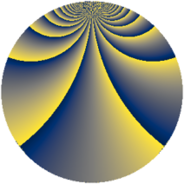# Properties

 Label 648.4.yLevel $648$ Weight $4$ Character orbit 648.y Rep. character $\chi_{648}(25,\cdot)$ Character field $\Q(\zeta_{27})$ Dimension $1458$ Sturm bound $432$

# Related objects

## Defining parameters

 Level: $$N$$ $$=$$ $$648 = 2^{3} \cdot 3^{4}$$ Weight: $$k$$ $$=$$ $$4$$ Character orbit: $$[\chi]$$ $$=$$ 648.y (of order $$27$$ and degree $$18$$) Character conductor: $$\operatorname{cond}(\chi)$$ $$=$$ $$81$$ Character field: $$\Q(\zeta_{27})$$ Sturm bound: $$432$$

## Dimensions

The following table gives the dimensions of various subspaces of $$M_{4}(648, [\chi])$$.

Total New Old
Modular forms 5904 1458 4446
Cusp forms 5760 1458 4302
Eisenstein series 144 0 144

## Trace form

 $$1458q + O(q^{10})$$ $$1458q + 1854q^{41} + 1026q^{45} - 594q^{47} - 2961q^{51} - 2862q^{53} - 2214q^{57} - 1449q^{59} + 1998q^{63} + 4266q^{65} + 3366q^{69} - 6345q^{89} - 5562q^{93} - 7938q^{95} + 5670q^{99} + O(q^{100})$$

## Decomposition of $$S_{4}^{\mathrm{new}}(648, [\chi])$$ into newform subspaces

The newforms in this space have not yet been added to the LMFDB.

## Decomposition of $$S_{4}^{\mathrm{old}}(648, [\chi])$$ into lower level spaces

$$S_{4}^{\mathrm{old}}(648, [\chi]) \cong$$ $$S_{4}^{\mathrm{new}}(81, [\chi])$$$$^{\oplus 4}$$$$\oplus$$$$S_{4}^{\mathrm{new}}(162, [\chi])$$$$^{\oplus 3}$$$$\oplus$$$$S_{4}^{\mathrm{new}}(324, [\chi])$$$$^{\oplus 2}$$